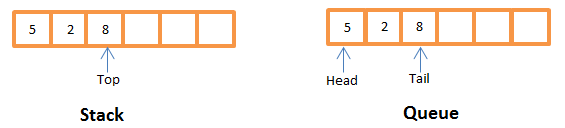# Introduction to Stack and Queue Data Structure and Analysis on Insert, Delete time

 Category: Data Structure And Algorithms Tags:Stack and Queue Code Files

## Introduction

When we code in any programming language we need to store data in different type of objects, sometimes list of objects, object of objects etc. How do we store the data and we must know how to design a data structure which should be efficient to manipulate it like time of insertion, deletion, search.

In this article we will learn, what is queue and stack, how to implement them with some access methods and then we will analyze their efficiency.

Stack: follows FILO (First In Last Out). Where one who comes first is served last. Insert and delete operations are held at same end.

Queue: follows FIFO (First In First Out), similar to any queue (i.e. at ticket counter). Where one who come first is served first.In above figure we can see stack is having only one pointer to perform operations but queue has two pointers where tail is used to insert and head is used to remove.

## Implementation

Stack: Implementation is given below:

```public class Stack
{
int top; //top pointer
int[] stackArr; //stack
public Stack(int stackSize)
{
top = 0;
stackArr = new int[stackSize];
}
/// <summary>
/// Inserts value on top of the stack
/// </summary>
/// <param name="value"></param>
public void Push(int value)
{
if (top < stackArr.Length)
{
stackArr[top] = value;
top++;
}
else
throw new Exception("Overflow");
}
/// <summary>
/// Removes and returns value from top
/// </summary>
/// <returns></returns>
public int Pop()
{
if (top >= 1)
{
top--;
return stackArr[top];
}
else
throw new Exception("Underflow");
}
/// <summary>
/// Returns actual number of element present in stack
/// </summary>
/// <returns></returns>
public int Count()
{
return top;
}
}
```

In stack we have implemented three methods Push, Pop and Count. Push is used to insert an element in stack, Pop is used to remove one and return that element from head, and Count returns the actual number of elements available in stack.

You can run this class to check the output like below:

```Stack stack = new Stack(10);
stack.Push(5);
stack.Push(3);
stack.Push(6);
stack.Pop();
stack.Push(4);
while(stack.Count() > 0)
{
Console.WriteLine(stack.Pop());
}
Console.ReadLine();
```

Output will be:

4

3

5

Queue: Implementation is given below:

```public class Queue
{
int head; //head pointer
int tail; //tail pointer
int[] queueArr; //queue
public Queue(int queueSize)
{
head = 0;
tail = 0;
queueArr = new int[queueSize];
}
/// <summary>
/// Adds element at end of the queue
/// </summary>
/// <param name="value"></param>
public void EnQueue(int value)
{
if (tail < queueArr.Length)
{
queueArr[tail] = value;
tail++;
}
else
throw new Exception("Overflow");
}
/// <summary>
/// Removes and returns element from head of the queue
/// </summary>
/// <returns></returns>
public int DeQueue()
{
if (head < tail)
{
int value = queueArr[head];
head++;
//if all the elements are removed, we can start from 0 to utilize the space
if (head == tail)
head = tail = 0;
return value;
}
else
throw new Exception("Underflow");
}
/// <summary>
/// Gives number of elements present in queue
/// </summary>
/// <returns></returns>
public int Count()
{
return (tail - head);
}
}
```

In queue we do implement 3 methods, where EnQueue places one element at end of the queue, DeQueue removes one and returns that element from the head of queue, and Count gives the number of actual elements present in queue.

You can run the above class and check output:

```Queue q = new Queue(10);
q.EnQueue(3);
q.EnQueue(6);
q.EnQueue(4);
int val = q.DeQueue();
q.EnQueue(7);
while (q.Count() > 0)
{
Console.WriteLine(q.DeQueue());
}
Console.ReadLine();
```

Output will be:

6

4

7

*We will get Exceptions in both stack and queue, where Underflow will be thrown when array is empty but asked to pop or dequeue one, and overflow occurs when array is full but asked to push or enqueue one.

## Analysis

Stack: In stack as seen in both methods Push and Pop we don't have any loops, to push or pop we need only O(1) time, no need to traverse the whole array because operation is happening on top of single end. So only O(1) time is required to push and pop, if we want to traverse or pop all the elements it will take O(n) time, where n is the number of elements in stack.

Queue: Same as stack, we need only O(1) to EnQueue and DeQueue the element because both operations are happening at individual ends. And traversing or removing all elements from queue will take O(n) time where n is the number of elements in queue.

 Like0 People
Last modified on 30 October 2018Nikhil Joshi Ceo & Founder at Dotnetlovers Atricles: 132 Questions: 9 Given Best Solutions: 9 *

## Comments:

No Comments Yet

You are not loggedin, please login or signup to add comments:

## Existing User

Login via:## New Userx